# Marathon Match 95 - Marathon Match 95

## Key Information

Register
Submit
The challenge is finished.

## Challenge Overview

### Problem Statement

You are given an image. Your task is to approximate it as closely as possible by starting with a black canvas of the same size as the image and drawing a series of semi-transparent filled circles on it one by one. The circles can intersect and don't have to fit on the canvas fully. When a pixel of one color is drawn on top of a pixel of another color, for each of the R, G, B components of the colors their values are added and divided by 2 to get the value of corresponding component of the resulting color.

### Implementation

Your code should implement a single method draw(int H, int[] pixels, int N).
• H is the height of the image. You can get the width of the image W as pixels.size() / H.
• pixels gives you the image itself: pixels[R*W+C] describes the color of pixel in row R and column C, encoded as an integer with 8 bits per color component in RGB order. You can decode color components as follows:
`int R = (pixel >> 16), G = (pixel >> 8) & 0xff, B = pixel & 0xff`
• N is the maximal number of circles you can use. You don't have to use all of them, but you don't get extra points for using less than N.
The method should return a int[] describing the circles you want to draw, in the order in which you want to draw them. Circle i should be described with four numbers:
• ret[4*i] is the row coordinate of the pixel which contains the center of the circle (must be between -1000 and H+999, inclusive).
• ret[4*i+1] is the column coordinate of the pixel which contains the center of the circle (must be between -1000 and W+999, inclusive).
• ret[4*i+2] is the radius of the circle (must be between 0 and 1000, inclusive).
• ret[4*i+3] is the color of the circle (in the same format as in the pixels array, must be between 0x000000 and 0xFFFFFF, inclusive).

### Scoring

For each test case we will calculate your raw score. If your solution produced an invalid return (invalid representation of circles or too many of them), raw score for this test case will be -1. Otherwise, the score will be the sum of absolute values of component-wise differences of colors of each pixel in the original image and in your approximation.

Your overall score will be calculated in the following way: for each test case where your score is not -1, you get 1 point for each competitor you beat on this test case (i.e., your score on a test case is less than this competitor's score) and 0.5 points for each competitor you tie with (a tie with yourself is not counted); finally, the sum of points is divided by (the number of competitors - 1), then multiplied by 1000000 and divided by the number of test cases.

### Tools

An offline tester is available here. You can use it to test/debug your solution locally. You can also check its source code for exact implementation of test case generation and score calculation. That page also contains links to useful information and sample solutions in several languages.

### Definition

 Class: CirclesMix Method: drawImage Parameters: int, int[], int Returns: int[] Method signature: int[] drawImage(int H, int[] pixels, int N) (be sure your method is public)

### Notes

-N is chosen randomly between 20 and 1000.
-Images used in tests were downloaded from random image at Wikipedia. Images which were not primarily photographic or photo-realistic, such as line drawings or photos of paintings, were discarded. Each dimension of each image will be between 250 and 800 pixels.
-The time limit is 20 seconds per test case (this includes only the time spent in your code). The memory limit is 1024 megabytes.
-There is no explicit code size limit. The implicit source code size limit is around 1 MB (it is not advisable to submit codes of size close to that or larger). Once your code is compiled, the binary size should not exceed 1 MB.
-The compilation time limit is 30 seconds. You can find information about compilers that we use and compilation options here.
-There are 10 example test cases and 100 full submission (provisional) test cases. There will be 1000 test cases in the final testing.
-The match is rated.

### Examples

0)

 `"1"`
```Returns: "seed = 1
"```
 H = 600 W = 600 N = 201)

 `"2"`
```Returns: "seed = 2
"```
 H = 600 W = 750 N = 1000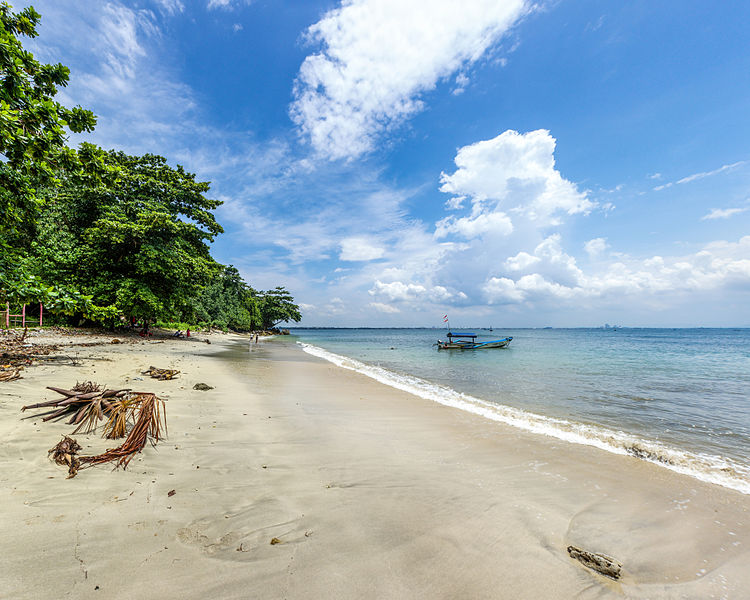2)

 `"3"`
```Returns: "seed = 3
"```
 H = 533 W = 800 N = 5553)

 `"4"`
```Returns: "seed = 4
"```
 H = 600 W = 400 N = 518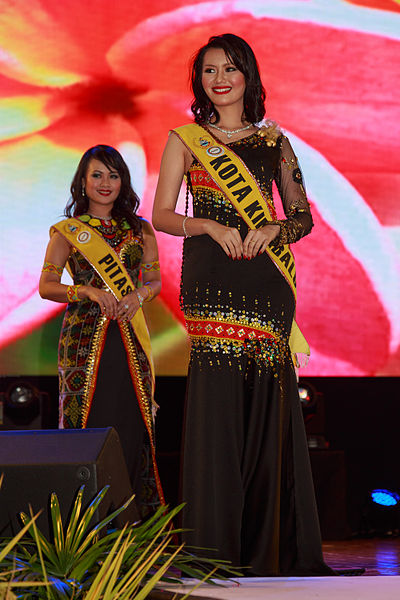4)

 `"5"`
```Returns: "seed = 5
"```
 H = 533 W = 800 N = 970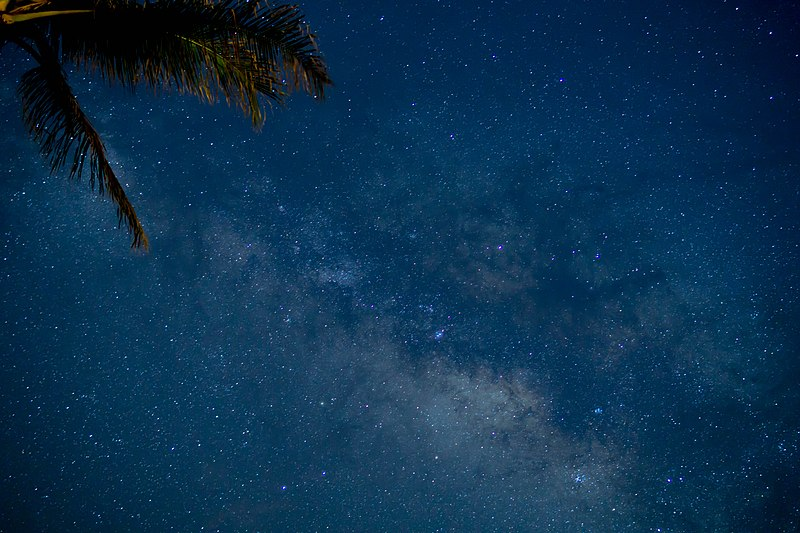5)

 `"6"`
```Returns: "seed = 6
"```
 H = 534 W = 800 N = 728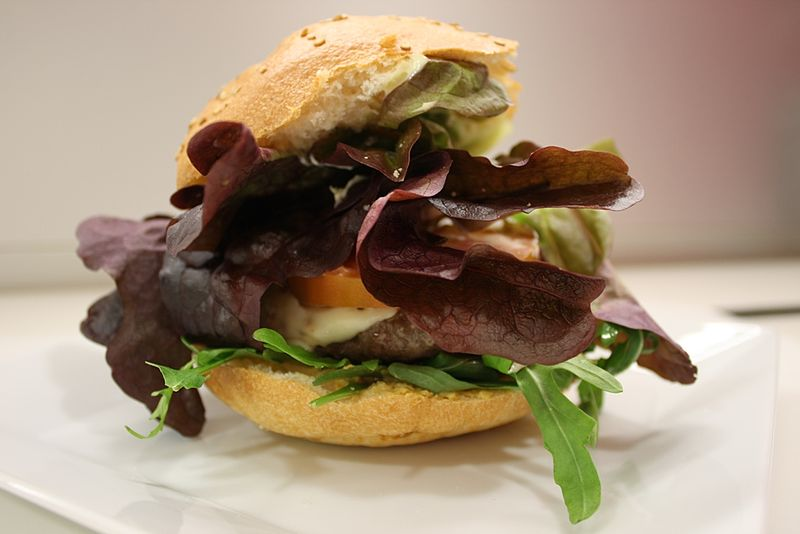6)

 `"7"`
```Returns: "seed = 7
"```
 H = 600 W = 800 N = 152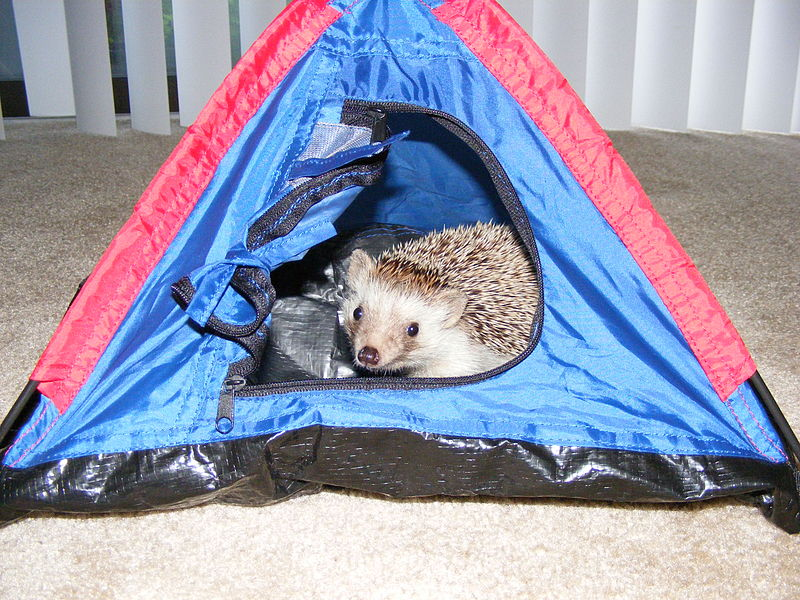7)

 `"8"`
```Returns: "seed = 8
"```
 H = 529 W = 800 N = 294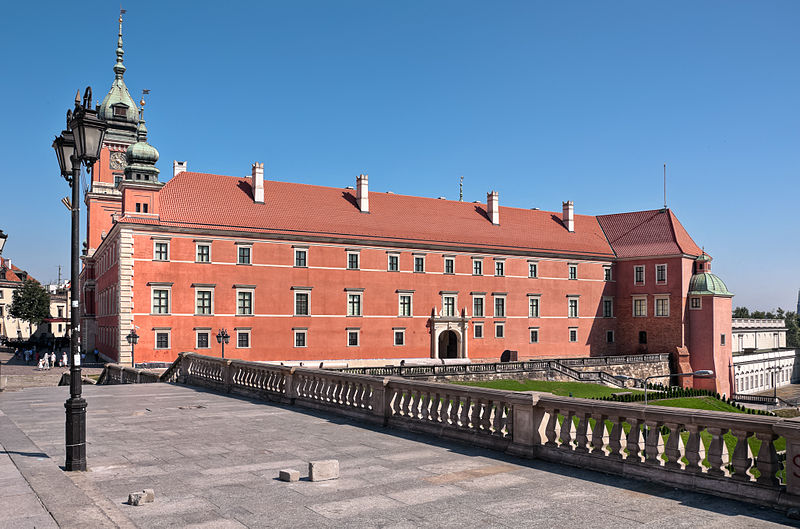8)

 `"9"`
```Returns: "seed = 9
"```
 H = 599 W = 400 N = 552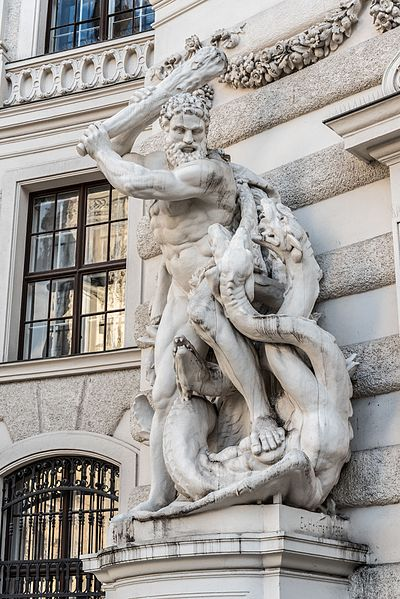9)

 `"10"`
```Returns: "seed = 10
"```
 H = 600 W = 800 N = 474This problem statement is the exclusive and proprietary property of TopCoder, Inc. Any unauthorized use or reproduction of this information without the prior written consent of TopCoder, Inc. is strictly prohibited. (c)2020, TopCoder, Inc. All rights reserved.

## Payments

Topcoder will compensate members in accordance with our standard payment policies, unless otherwise specified in this challenge. For information on payment policies, setting up your profile to receive payments, and general payment questions, please refer to ‌Payment Policies and Instructions.

## LEARN:

Topcoder Challenges Explained